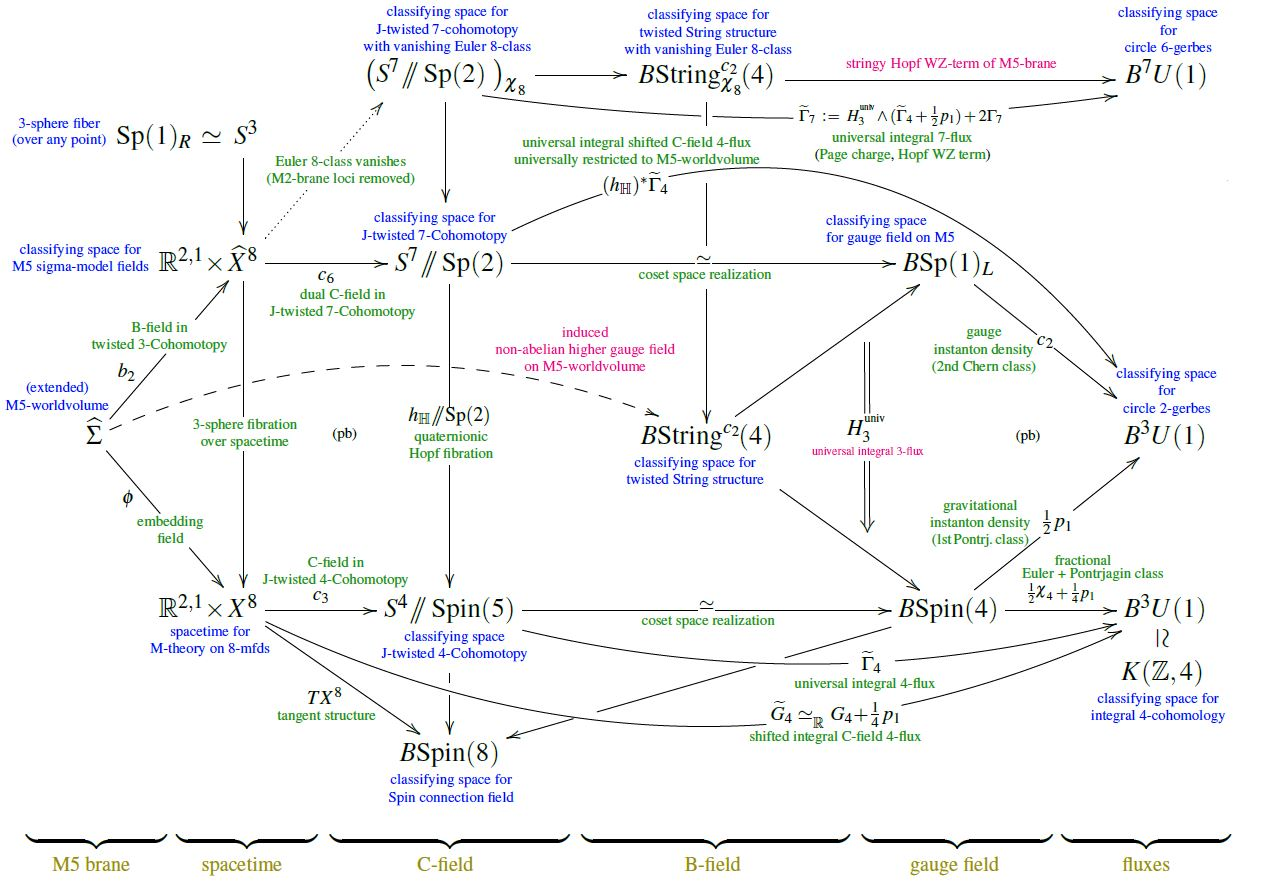# Schreiber Twisted Cohomotopy implies twisted String structure on M5-branes

An article that we have written:

Abstract. We show that charge-quantization of the M-theory C-field in J-twisted Cohomotopy theory (Hypothesis H) implies emergence of a higher Sp(1)-gauge field on single heterotic M5-branes, which exhibits worldvolume twisted string structure.(this homotopy-commutative diagram became the logo of M-Theory and Mathematics 2023)

Errata

• In the expression for $2 \widetilde \Gamma_7$ on the first and last page the term “$\widetilde \Gamma + \tfrac{1}{2}p_1$” should read just “$\widetilde \Gamma_4$”.

(There are two alternative ways of defining $\widetilde \Gamma$ as $\tfrac{1}{2}\chi \pm \tfrac{1}{4}p_1$, which differ by that summand $\tfrac{1}{2}p_1$. But with the convention that we ended up using on p. 4, this conversion summand is absent.)

Followups:

Exposition and talk notes on Hypothesis H:

Related articles on Hypothesis H:

Last revised on June 26, 2023 at 07:04:47. See the history of this page for a list of all contributions to it.